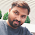### Problem

Write an efficient algorithm that searches for a value in an m x n matrix. This matrix has properties:
1) Integers in each row are sorted from left to right. 2) The first integer of each row is greater than the last integer of the previous row.

### Example

Input ::
[
[1,   3,  5,  7],
[10, 11, 16, 20],
[23, 30, 34, 50]
]
Given target = 3, return true.

### JavaScript Code

```function searchMatrix(matrix, target) {
if(matrix==null || matrix.length==0 || matrix.length==0)
return false;

var m = matrix.length;
var n = matrix.length;

var start = 0;
var end = m*n-1;

while(start<=end){
var mid=parseInt((start+end)/2);
var midX=parseInt(mid/n);
var midY=mid%n;

if(matrix[midX][midY]==target)
return true;

if(matrix[midX][midY]<target){
start=mid+1;
} else {
end=mid-1;
}
}

return false;
}
```

#### 1 comment:

1.You are awesome really :)

## BlogrollSrinivas Dasari find me on facebook follow me on twitter